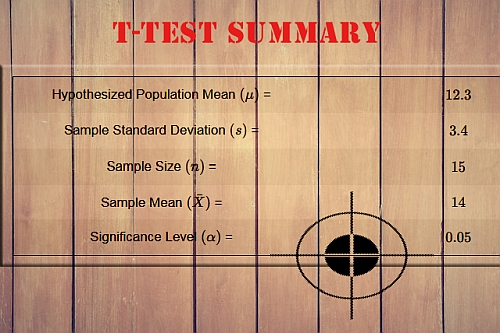# T-test for One Population Mean

Instructions: This calculator conducts a t-test for one population mean ($$\sigma$$), with unknown population standard deviation ($$\sigma$$), for which reason the sample standard deviation (s) is used instead. Please select the null and alternative hypotheses, type the hypothesized mean, the significance level, the sample mean, the sample standard deviation, and the sample size, and the results of the t-test will be displayed for you:

Ho: $$\mu$$ $$\mu_0$$
Ha: $$\mu$$ $$\mu_0$$Hypothesized Mean ($$\mu_0$$)Sample Mean ($$\bar X$$)Sample St. Deviation ($$s$$)Sample Size (n)Significance Level ($$\alpha$$)

## How to use this t-test calculator for One Sample

More about the t-test for one mean so you can better interpret the results obtained by this solver: A t-test for one mean is a hypothesis test that attempts to make a claim about the population mean ($$\sigma$$). This t-test, unlike the z-test, does not need to know the population standard deviation $$\sigma$$.### How to Conduct a T-test for One Population Mean?

The test has two complementary hypotheses, the null and the alternative hypothesis. The null hypothesis is a statement about the population mean, under the assumption of no effect, and the alternative hypothesis is the complementary hypothesis to the null hypothesis. The main properties of a one sample t-test for one population mean are:

• For a t-test for one mean, the sampling distribution used for the t-test statistic (which is the distribution of the test statistic under the assumption that the null hypothesis is true) corresponds to the t-distribution, with n-1 degrees of freedom (instead of being the standard normal distribution, as in the case of a z-test for one mean)

• Depending on our knowledge about the "no effect" situation, the t-test can be two-tailed, left-tailed or right-tailed

• The main principle of hypothesis testing is that the null hypothesis is rejected if the test statistic obtained is sufficiently unlikely under the assumption that the null hypothesis is true

• The p-value is the probability of obtaining sample results as extreme or more extreme than the sample results obtained, under the assumption that the null hypothesis is true

• In a hypothesis tests there are two types of errors. Type I error occurs when we reject a true null hypothesis, and the Type II error occurs when we fail to reject a false null hypothesis

### How to Compute the t-statistic for one sample?

So, what is the one sample t test formula? In this case, for this t-test formula for the t-statistic is

$t = \frac{\bar X - \mu_0}{s/\sqrt{n}}$

The null hypothesis is rejected when the t-statistic lies on the rejection region, which is determined by the significance level ($$\alpha$$) the type of tail (two-tailed, left-tailed or right-tailed) and the number of degrees of freedom $$df = n - 1$$### What happens with the t-test when I have 2 samples

Notice that this is a one sample t test calculator. If instead you need to compare two means, you should use a t-test for independent samples , instead.

In a similar way, you may have two samples but they are paired, matched or repeated, which case the appropriate tool to use is this paired t test calculator, when that is the case.

### Decision for a one sample t-test

How do you make a decision on a one-sample t-test? First, you need to know the t-statistic, which we call $$t_{obs}$$, and the degrees of freedom df, so that you can compute the p-value.

The process of calculation of the p-value will depend on the type of tails defined. For a two-tailed test, the p-value is computed as $$p = \Pr(|t_{df}| > |t_{obs}|)$$. Then, for a left-tailed test, the p-value is computed as $$p = \Pr(t_{df} < t_{obs})$$, and for a right-tailed test, the p-value is computed as $$p = \Pr(t_{df} > t_{obs})$$.

### One sample t-test example

A vendor has records showing that the average customer spends $80 dollars in her store on average, but as of recent, she feels that amount has increased. She collects a random sample of n = 30 customers, and she finds that the mean amount spent on the store was$85.4, with a sample standard deviation of \$12.4. Does she have enough evidence to claim that the average spent on her store has increased significantly, at the .05 significance level?

Solution:

The following information has been provided:

 Hypothesized Population Mean $$(\mu)$$ = $$80$$ Sample Standard Deviation $$(s)$$ = $$12.4$$ Sample Size $$(n)$$ = $$30$$ Sample Mean $$(\bar X)$$ = $$85.4$$ Significance Level $$(\alpha)$$ = $$0.05$$

(1) Null and Alternative Hypotheses

The following null and alternative hypotheses need to be tested:

$\begin{array}{ccl} H_0: \mu & = & 80 \\\\ \\\\ H_a: \mu & > & 80 \end{array}$

This corresponds to a right-tailed test, for which a t-test for one mean, with unknown population standard deviation, using the sample standard deviation, will be used.

(2) Rejection Region

Based on the information provided, the significance level is $$\alpha = 0.05$$, and the critical value for a right-tailed test is $$t_c = 1.699$$.

The rejection region for this right-tailed test is $$R = \{t: t > 1.699\}$$

(3) Test Statistics

The t-statistic is computed as follows:

$\begin{array}{ccl} t & = & \displaystyle \frac{\bar X - \mu_0}{s/\sqrt{n}} \\\\ \\\\ & = & \displaystyle \frac{ 85.4 - 80}{ 12.4/\sqrt{ 30}} \\\\ \\\\ & = & 2.385 \end{array}$

(4) Decision about the null hypothesis

Since it is observed that $$t = 2.385 > t_c = 1.699$$, it is then concluded that the null hypothesis is rejected.

Using the P-value approach: The p-value is $$p = 0.0119$$, and since $$p = 0.0119 < 0.05$$, it is concluded that the null hypothesis is rejected.

(5) Conclusion

It is concluded that the null hypothesis Ho is rejected. Therefore, there is not enough evidence to claim that the population mean $$\mu$$ is greater than 80, at the $$\alpha = 0.05$$ significance level.

Confidence Interval

The 95% confidence interval is $$80.77 < \mu < 90.03$$.

Graphically